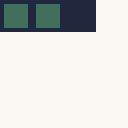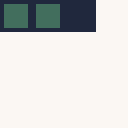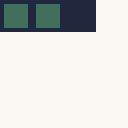# Moving the Snake

To move the snake, you have to change the X and Y position of every body-part of the snake.

For this, you can start at the end of the body and give this part the values of the part ahead. Since the final piece to get its values "stolen" is the head, the head seems to disappear for very brief moment.

After this is done, you simply move the head in the direction of the snake. Since all of this happens, before the snake is rendered, it appears as a fluid motion.

This is how the logic looks like:Keep in mind: This is not what the player sees. This is:## Moving the Body#

To achieve the first step (moving the body, excluding the head), a simple loop is all you need:

``    update(): void {        let body = this.body;        for (let i = body.length - 1; i > 0; i--) {            unchecked(body[i].x = body[i - 1].x)            unchecked(body[i].y = body[i - 1].y)        }    }``

Don't forget to call the new function in the main-loop:

``export function update(): void {    snake.update()    snake.draw()}``

Now if you execute this, you'd notice that you can't see much. In fact, you might see the snake for a short moment before the head is all that's left.This isn't hard either. Simple add the add the direction to the current head. And then make sure the head stays within the boundaries:

``````    update(): void {        let body = this.body;        for (let i = body.length - 1; i > 0; i--) {            unchecked(body[i].x = body[i - 1].x)            unchecked(body[i].y = body[i - 1].y)        }
body.x = (body.x + this.direction.x) % 20        body.y = (body.y + this.direction.y) % 20
if (body.x < 0) {            body.x = 19        }        if (body.y < 0) {            body.y = 19        }    }``````

That's it. Now you should see the snake running from left to right. Maybe a little too fast, though.## Slowing Down#

By default WASM-4 runs at 60 FPS. This means your little snake moves 60 fields in each second. That is 3 times the whole screen. There are several ways to slow the snake down.

The easiest way is probably to count the frames and update the snake only every X frames.

For this, you'd need a new variable. You can call it whatever you like, just be sure you know what its purpose is.

``const snake = new Snake()let frameCount = 0``

This variable in main.ts keeps track of all frames so far. Just increase its value in the main-update function:

``````export function update(): void {    frameCount++
snake.update()
snake.draw()}``````

Now all you need is to check if the passed frames are dividable by X:

``````export function update(): void {    frameCount++
if (frameCount % 15 == 0) {        snake.update()    }
snake.draw()}``````

That's it. Your snake should be quite a bit slower now. This reduces the snake from 60 units per second to 4 units per second (60/15 = 4).